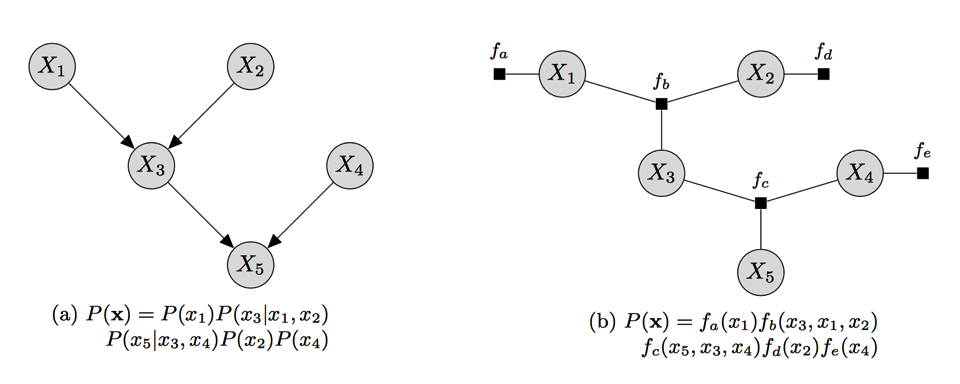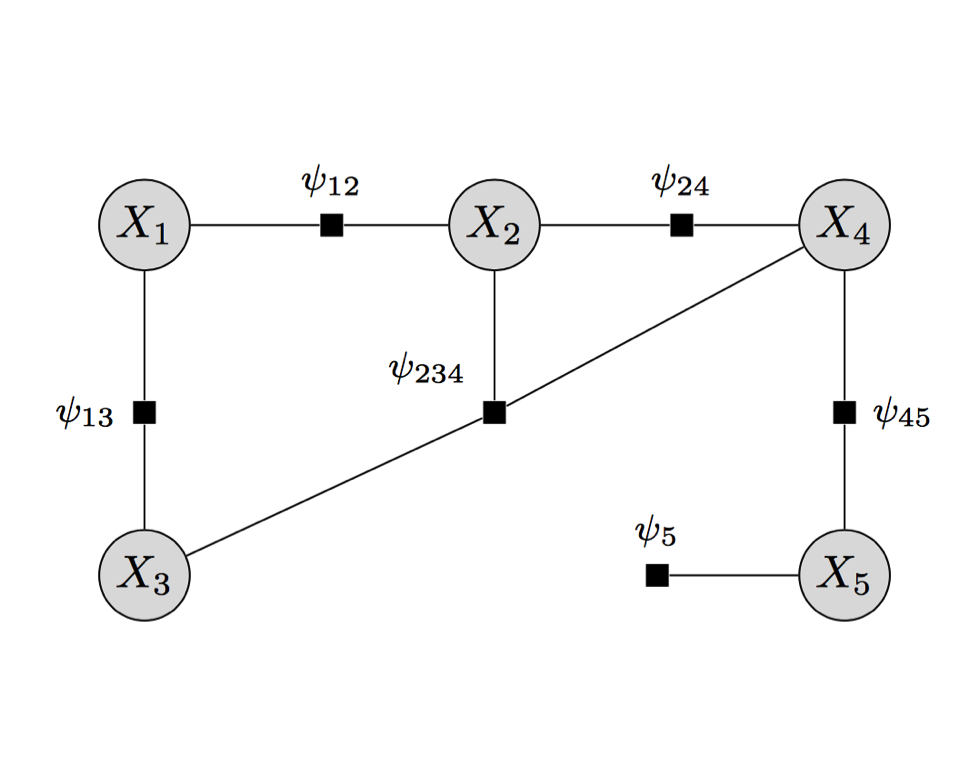September 27, 2016

## Course Logistics

• Office hour: 2-3pm on Tuesdays or by appointment
• New office: 4623 SPH-I (within Suite 4605)
• Homework 1 due 11:59pm on October 10, 2016 (Questions?)

## Inference Problems: Three Types of Tasks

• Marginal probabilities. Compute marginals of variables (given model parameters $$\mathbf{\theta}$$): $$p(x_i\mid \mathbf{\theta})=\sum_{\mathbf{x}': x_i'=x_i}p(\mathbf{x}'\mid \mathbf{\theta}).$$ (Or conditional probabilities: posterior distribution)

• Partition function. For a Gibbs distribution representable as normalized products of factors: $$p(\mathbf{x})=\frac{1}{Z}\prod_{C\in\mathcal{C}}\psi_{C}(\mathbf{x}_C)$$ with respect to a graph $$\mathcal{G}$$ with cliques $$\mathcal{C}$$, compute $Z(\mathbf{\theta})=\sum_{\mathbf{x}}\prod_{C\in\mathcal{C}}\psi_{C}(\mathbf{x}_C)$

• Maximum A Posteriori (MAP) Inference. Compute the variable configuration with the hightest probability: $$\hat{\mathbf{x}}=\arg \max_{\mathbf{x}}p(\mathbf{x}\mid \mathbf{\theta}),$$ where $$p$$ is a generic notation for joint or conditional distributions with unobserved variables $$\mathbf{X}=\mathbf{x}$$.

## Compute Marginal Probabilities

• Let $$\mathbf{X}=\{X_1,\ldots,X_d\}$$ denote the set of variable nodes, each of which can range from $$1$$ to $$k$$; $$F=\{\psi_{\alpha_1},\ldots, \psi_{\alpha_s}\}$$ denote the set of factor nodes, where $$\alpha_1, \ldots, \alpha_s \subset \{1,\ldots, d\}$$.
• Compute the marginal probability naively, we do: $p(X_1=x_1)=\frac{1}{Z}\sum_{x_2,\ldots, x_d}\prod_{i=1}^s \psi_{\alpha_i}(\mathbf{x}_{\alpha_i})$

• Computational complexity: $$\mathcal{O}(k^{d-1})$$ additions of values read from the probability table, one row for each configuration $$X_2=x_2,\ldots,X_d=x_d$$.

• Instead, we choose an ordering $$\mathcal{I}$$ among $$\{X_1,\ldots,X_d\}$$ and utilize the factorization of $$p(\mathbf{x})$$. (Variable Elimination)

## Factor Graph

• Explicitly encondes the relations among a subset of variables, which sometimes are not possible by clique potential parameterization (see an example on the next slide).
• Supports the inference/computational techniques
• Unifies directed and undirected graphical models
• Example (whiteboard)## Factor Graph: A Refinement

• Example:
• $$\mathcal{H}=(V,E)$$: complete (fully connected) undirected graph with vertices $$V$$ and edges $$E$$. $$|V|=d$$
• $$P$$: positive distribution over $$d$$ binary nodes; has only pairwise potentials (pairwise dependencies cannot be conditioned away).
• $$P$$ is Markov to $$\mathcal{H}$$, but not Markov to any graph missing even one edge.
• The graph $$\mathcal{H}$$ can only guide use to use the maximal clique potential parametrization. For a omplete graph, we use a single, large potential $$\psi(X_1,\ldots,X_d)$$, which requires $$2^d$$ parameters.
• However, $$P$$ by definition can be represented in a Gibbs distribution only with pairwise potentials $$\psi_{{j}{j'}}(X_j,X_{j'})$$, which requires $$4{d \choose 2}$$ parameters
• When $$d=10$$, $$2^d=1,024$$, much bigger than $$4{d \choose 2}=180$$.

## Variable Eliminations for $$p(X_1=x_1)$$ for $$p(X_1,\ldots, X_d)$$

1. Initialize an active list of potentials as read off from the factor graph.
2. Choose an ordering $$\mathcal{I}$$ for the variables in which $$x_1$$ appears last
3. For each $$i=1,\ldots,d-1$$, let $$\mathcal{I}_i=j$$, do the following:
• Find all potentials in the currently active list that reference $$x_j$$ and remove them from the active list
• Let $$\phi_{j}(X_{T_j})$$ denote the product of these potentials, with $$T_j$$ being the union of nodes appearing in the active potentials referencing $$X_j$$.
• Calculate $$\tau_i(x_{T_j-X_j})=\sum_{x_j}\phi_{j}(x_{T_j})$$
• Place $$\tau_i(x_{T_j-X_j})$$ on the active list as one potential function
4. Finally, we obtain $$p(x_1)=\frac{1}{Z}\tau_{d-1}(x_1)$$

## Example: Variable Elimination for Factor Graph

• Let $$X_i\in \{1,\ldots,k\}$$.
• Describe how you would compute $$p(X_1=x_1)$$.
• Computational complexity of variable elimination: $$\mathcal{O}((d-1)k^r)$$, where $$d$$ is the number of variables, $$k$$ is the maximum value a variable can take and $$r$$ is the number of variables participating in the largest intermediate "factor".
• Finding a good ordering can reduce the computational complexity.## Comment

• Next Lecture: Belief propagation (Sum-Product Algorithm on Polytrees)

• Required reading for the week: Chapter 9. Koller and Friedman (2009)